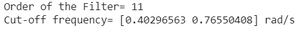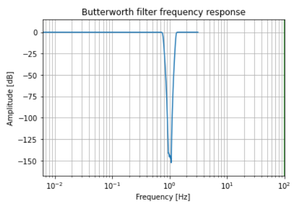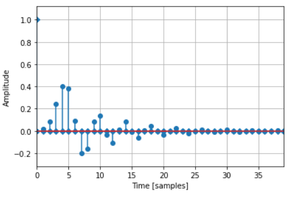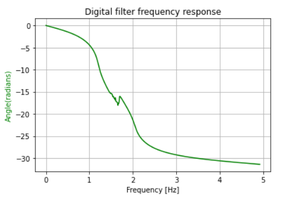Digital Band Reject Butterworth Filter in Python

• Last Updated : 03 Jan, 2021

In this article, we are going to discuss how to design a Digital Band Reject Butterworth Filter using Python. The Butterworth filter is a type of signal processing filter designed to have a frequency response as flat as possible in the pass band. Let us take the below specifications to design the filter and observe the Magnitude, Phase & Impulse Response of the Digital Butterworth Filter.

What is Digital Bandreject Filter?

A band-pass filter is a filter that passes frequencies within a range and rejects frequencies outside that range.

How it’s different from Highpass, Lowpass & Bandpass:

The main difference can be spotted by observing the magnitude response of the Band Pass Filter. In the Band-Reject filter, all the signals between the specified frequency range get rejected by the filter.

The specifications are as follows:

• The sampling rate of 12 kHz.
• Pass band edge frequencies are 2100 Hz & 4500 Hz.
• Stop band edge frequencies are 2700 Hz & 3900 Hz.
• Pass band ripple of 0.6 dB.
• Minimum stop band attenuation of 45 dB.

We will plot the magnitude, phase, and impulse response of the filter.

Step-by-step Approach:

Before starting, first, we will create a user-defined function to convert the edge frequencies, we are defining it as the convert() method.

Python3

 # explicit function to convert# edge frequencies    def convertX(f_sample, f):    w = []      for i in range(len(f)):        b = 2*((f[i]/2) / (f_sample/2))        w.append(b)      omega_mine = []      for i in range(len(w)):        c = (2/Td)*np.tan(w[i]/2)        omega_mine.append(c)      return omega_mine

Step 1: Importing all the necessary libraries.

Python3

 # import required modulesimport numpy as npimport matplotlib.pyplot as pltfrom scipy import signalimport math

Step 2: Define variables with the given specifications of the filter.

Python3

 # Specifications of Filter  # sampling frequencyf_sample = 12000  # pass band frequencyf_pass = [2100, 4500]  # stop band frequencyf_stop = [2700, 3900]  # pass band ripplefs = 0.5  # Sampling TimeTd = 1  # pass band rippleg_pass = 0.6  # stop band attenuationg_stop = 45

Step 3: Building the filter using signal.buttord() function.

Python3

 # Conversion to prewrapped analog# frequencyomega_p = convertX(f_sample, f_pass)omega_s = convertX(f_sample, f_stop)  # Design of Filter using signal.buttord# functionN, Wn = signal.buttord(omega_p, omega_s,                       g_pass, g_stop,                       analog=True)    # Printing the values of order & cut-off frequency# N is the orderprint("Order of the Filter=", N)  # Wn is the cut-off freq of the filterprint("Cut-off frequency= {:} rad/s ".format(Wn))    # Conversion in Z-domain  # b is the numerator of the filter & a is# the denominatorb, a = signal.butter(N, Wn, 'bandpass', True)z, p = signal.bilinear(b, a, fs)  # w is the freq in z-domain & h is the# magnitude in z-domainw, h = signal.freqz(z, p, 512)

Output:Step 4: Plotting the Magnitude Response.

Python3

 # Magnitude Responseplt.semilogx(w, 20*np.log10(abs(h)))plt.xscale('log')plt.title('Butterworth filter frequency response')plt.xlabel('Frequency [Hz]')plt.ylabel('Amplitude [dB]')plt.margins(0, 0.1)plt.grid(which='both', axis='both')plt.axvline(100, color='green')plt.show()

Output:Step 5: Plotting the Impulse Response.

Python3

 # Impulse Responseimp = signal.unit_impulse(40)c, d = signal.butter(N, 0.5)response = signal.lfilter(c, d, imp)  plt.stem(np.arange(0, 40), imp, markerfmt='D', use_line_collection=True)plt.stem(np.arange(0, 40), response, use_line_collection=True)plt.margins(0, 0.1)  plt.xlabel('Time [samples]')plt.ylabel('Amplitude')plt.grid(True)plt.show()

Output:Step 6: Plotting the Phase Response.

Python3

 # Frequency Responsefig, ax1 = plt.subplots()ax1.set_title('Digital filter frequency response')ax1.set_ylabel('Angle(radians)', color='g')ax1.set_xlabel('Frequency [Hz]')  angles = np.unwrap(np.angle(h))  ax1.plot(w/2*np.pi, angles, 'g')ax1.grid()ax1.axis('tight')plt.show()

Output:Below is the complete program based on the above approach:

Python3

 # import required modulesimport numpy as npimport matplotlib.pyplot as pltfrom scipy import signalimport math  # explicit function to convert# edge frequenciesdef convertX(f_sample, f):    w = []      for i in range(len(f)):        b = 2*((f[i]/2)/(f_sample/2))        w.append(b)      omega_mine = []      for i in range(len(w)):        c = (2/Td)*np.tan(w[i]/2)        omega_mine.append(c)      return omega_mine  # Specifications of Filter# sampling frequencyf_sample = 12000  # pass band frequencyf_pass = [2100, 4500]  # stop band frequencyf_stop = [2700, 3900]  # pass band ripplefs = 0.5  # Sampling TimeTd = 1  # pass band rippleg_pass = 0.6  # stop band attenuationg_stop = 45  # Conversion to prewrapped analog# frequencyomega_p = convertX(f_sample, f_pass)omega_s = convertX(f_sample, f_stop)  # Design of Filter using signal.buttord# functionN, Wn = signal.buttord(omega_p, omega_s, g_pass,                       g_stop, analog=True)  # Printing the values of order & cut-off frequency# N is the orderprint("Order of the Filter=", N)  # Wn is the cut-off freq of the filterprint("Cut-off frequency= {:} rad/s ".format(Wn))    # Conversion in Z-domain  # b is the numerator of the filter & a is# the denominatorb, a = signal.butter(N, Wn, 'bandpass', True)z, p = signal.bilinear(b, a, fs)  # w is the freq in z-domain & h is the# magnitude in z-domainw, h = signal.freqz(z, p, 512)  # Magnitude Responseplt.semilogx(w, 20*np.log10(abs(h)))plt.xscale('log')plt.title('Butterworth filter frequency response')plt.xlabel('Frequency [Hz]')plt.ylabel('Amplitude [dB]')plt.margins(0, 0.1)plt.grid(which='both', axis='both')plt.axvline(100, color='green')plt.show()  # Impulse Responseimp = signal.unit_impulse(40)c, d = signal.butter(N, 0.5)response = signal.lfilter(c, d, imp)  plt.stem(np.arange(0, 40), imp,         markerfmt='D',         use_line_collection=True)  plt.stem(np.arange(0, 40), response,         use_line_collection=True)  plt.margins(0, 0.1)  plt.xlabel('Time [samples]')plt.ylabel('Amplitude')plt.grid(True)plt.show()  # Frequency Responsefig, ax1 = plt.subplots()ax1.set_title('Digital filter frequency response')ax1.set_ylabel('Angle(radians)', color='g')ax1.set_xlabel('Frequency [Hz]')  angles = np.unwrap(np.angle(h))  ax1.plot(w/2*np.pi, angles, 'g')ax1.grid()ax1.axis('tight')plt.show()

Output:My Personal Notes arrow_drop_up